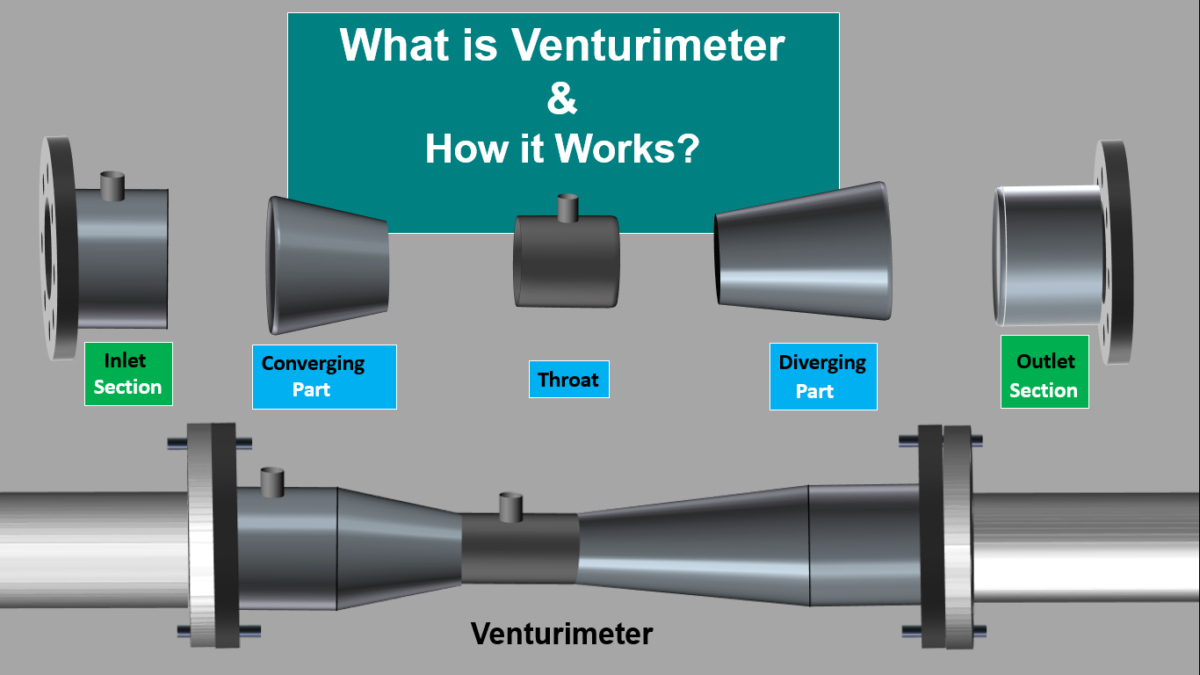# Venturimeter Working Principle| What Is a Venturimeter | Parts of VenturimeterVenturimeter is a device which is installed in a pipeline to measure the flow rate of Gas or Liquid flowing through a pipe. Venturimeter is attached to a pipe using flanges, so that the fluid which is flowing through the pipe also flows through the Venturimeter.

Venturimeter works by measuring the difference in pressure at two different locations. The pressure difference is created by introducing a Constriction in the path of the fluid flowing through the pipe, causing the fluid to flow faster. This pressure difference is then used to calculate the FLOW RATE.

Parts of a Venturimeter

Venturimeter is a device which does not have any moving part. It is mainly made up of three parts- Converging part, Throat and Diverging part. These Three parts are arranged in a systematic order.

Now let’s discuss about the Converging Part.

It is a Region of the Venturimeter where the Cross Section Emerges into a Conical Shape. which means In this part the Cross-sectional area decreases from beginning to the End.
This section is Connected to inlet section on one end and Cylindrical throat on the other end.
The inlet section of the Venturimeter is of the same diameter as that of the pipeline in which the venturimeter is installed.

The second part is the Cylindrical Throat

It is the middle part of the venturimeter through which the fluid passes after converging in the converging part.
Generally diameter of the throat is half the diameter of the Pipe.
The Diameter of the Throat remains the same throughout its Length. One End of the Throat is connected to the Converging part and the other end is connected to the Diverging part.

The last part is the Diverging part.

It is the end of the Venturimeter. On one side it is attached to the throat and on the other side it is attached to the outlet part, which is connected to the pipe.

In Diverging part the Cross sectional area gradually increases. Length of converging part is shorter than the Length of the Divergent part.
There are two pressure Tappings or holes in the walls of the venturimeter for pressure measurement.
Venturimeter works by measuring the difference in pressure at these two Pressure Tappings.

Working Principle of Venturimeter.

Venturimeter Works on the Principle of Continuity Equation and Bernoulli’s theorem.
According to Continuity equation if the cross sectional area of a pipe decreases then the velocity of the fluid flowing through it increases.

Let’s consider the case of a garden hose used for watering the plants. when a finger is placed at the end of this hose the cross section of the hose decreases, then the speed or velocity of the water flowing out Increases.

Bernoulli’s theorem states that, For a flowing fluid, An increase in speed of fluid occurs with a decrease in pressure of the fluid. which means if we know how much pressure of fluid is decreased then we can find the measure by which the Speed of fluid has increased.

When this Continuity Equation and Bernoulli’s Theorem is applied on a Venturimeter we can find that, the Constriction introduced by the Venturimeter will increase the velocity of the fluid and this increase in Velocity will in turn reduce the Pressure of the Fluid.

How a Venturimeter Works?

The venturimeter is placed in a pipe carrying the fluid whose flow rate is to be measured.
Venturimeter has name plate on it which has a flow direction arrow according to which the Venturimeter needs to be installed in the pipeline.

The fluid which flows through the pipe now flows through the Venturimeter. As the fluid enters the inlet section, first it reaches the Converging part.

Due to the shape of this part the fluid starts to Converge as the Cross sectional area of this part is decreasing.

According to the continuity equation if the cross Sectional area decreases, then the velocity of the fluid Increases and According to Bernoulli’s theorem if the Velocity of the fluid increases, then the pressure has to Decrease, So the velocity of the fluid increases and the pressure of the fluid decreases.

After Converging part the fluid then Enters the middle part that is the Throat, which is of Constant Diameter. Since the Diameter is Constant the velocity of the flowing fluid does not change and also the pressure of the fluid does not change in the Throat.

A Pressure tapping is provided at the Throat and another Tapping is provided near the Inlet part before the fluid reaches the Convergent part.

Tapping’s are holes made on the walls of the venturimeter to measure Pressure. The high Pressure Tapping is at the Inlet Section which will give the Pressure of the fluid Flowing through the pipe, while the low Pressure Tapping is at the middle of the throat section which will give the pressure of the fluid after Converging.

The difference in pressure between these two tapping positions is measured using a manometer or a
Differential pressure gauge.
This Pressure difference is then used to Calculate the Flow Rate of the Fluid.
After the fluid crosses the throat section it passes through a Diverging part. The purpose of Diverging part is to Recover the pressure which was lowered by the Convergent Part.

In order to measure the flow rate when the fluid reaches the divergent part as the cross-sectional area is increasing the velocity of the flowing fluid Decreases and the Pressure Increases.